# “Trust, but verify” SPICE model accuracy, part 6: voltage noise and current noise

Previous installments of this blog post series discussed the need to verify SPICE model accuracy and how to measure common-mode rejection ratio (CMRR), offset voltage versus common-mode voltage (Vos vs. Vcm), slew rate (SR), open-loop output impedance (Zo), input offset voltage (Vos) and open-loop gain (Aol). In this sixth and final installment, I’ll cover operational amplifier (op amp) noise, including voltage noise and current noise.

Noise is simply an unwanted signal, usually random in nature, that when combined with your desired signal results in an error. All op amps, as well as certain other circuit elements like resistors and diodes, generate some amount of intrinsic – or internal – noise. In analog circuits, it’s critical to confirm that the noise level is low enough to obtain a clear measurement of your desired output signal. Figure 1 shows an example of input voltage, ideal output voltage and output voltage with noise for a circuit with gain of 3V/V.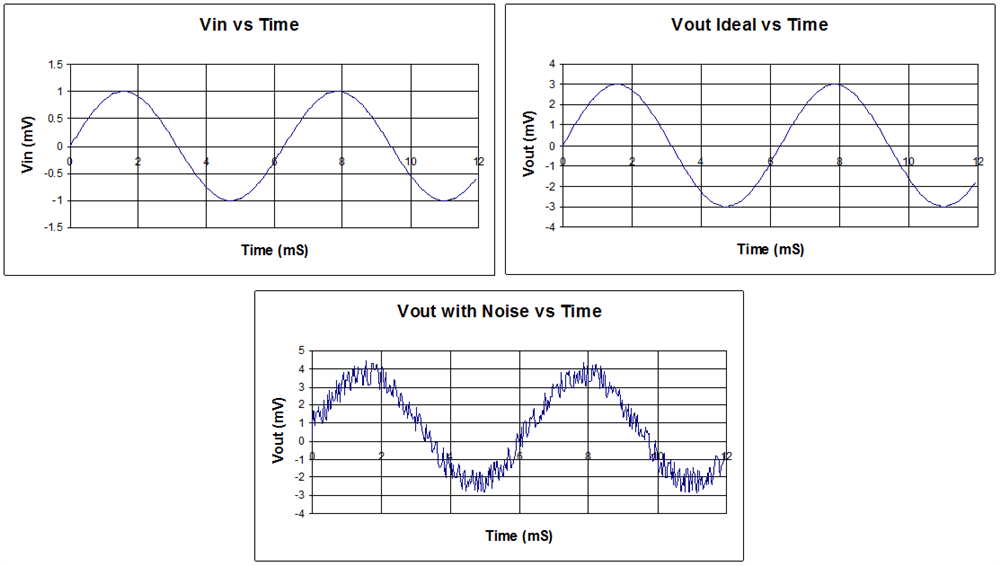Figure 1: Noise example

With an accurate model, predicting the noise performance of an op amp circuit becomes quite straightforward. This is very appealing to most engineers, as calculating noise by hand can be cumbersome and difficult.

Input voltage noise density

The voltage noise of an op amp is usually given as input voltage noise density (en) in nanovolts per square root hertz (nV/√Hz), which quantifies how much noise voltage the op amp generates at its input pins for any given frequency. To measure en, configure the op amp as a unity gain buffer with its noninverting input connected to an AC source Vin. Figure 2 shows the recommended test circuit.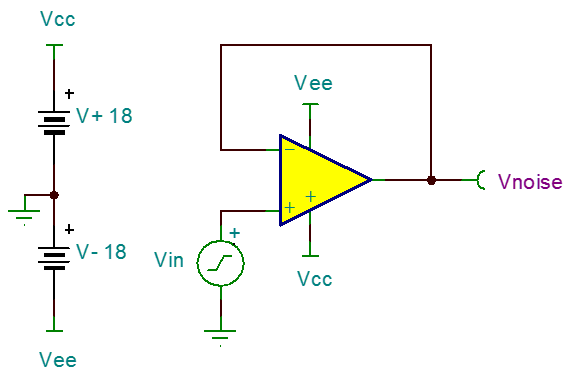Figure 2: Input voltage noise density test circuit

Let’s use this circuit to measure the en of the OPA1692, a low-noise amplifier from TI. Simply run a noise analysis over the desired frequency range and measure the noise level at node Vnoise with respect to Vin.

In this case, the simulated en matches perfectly with the data-sheet spec, shown in Figure 3.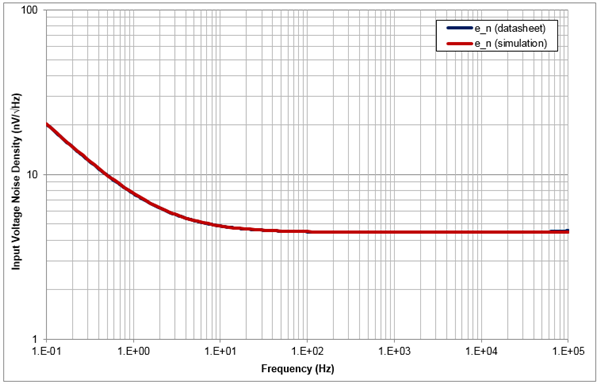Figure 3: OPA1692 en result

Input current noise density

Op amps also generate noise currents at their input pins, called input current noise density (in) and typically given in femtoamperes per square root hertz (fA/√Hz). You can measure this in a similar way to en, but you will need to perform a simple trick. Some simulators have trouble measuring noise in terms of current, so a current-controlled voltage source converts the current flowing into the noninverting input pin into a voltage. Figure 4 shows the recommended test circuit.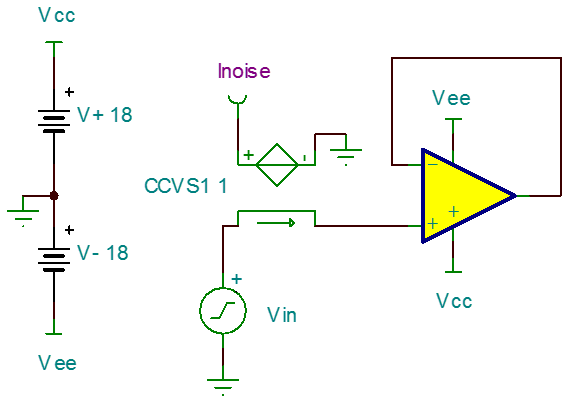Figure 4: Input current noise density test circuit

Let’s use this circuit to measure the in of the OPA1692. Run a noise analysis over the desired frequency range and measure the noise level at node Inoise with respect to Vin. Keep in mind that the resulting plot will have converted amps to volts due to current-controlled voltage source (CCVS1). Figure 5 shows the results after converting back to amperes.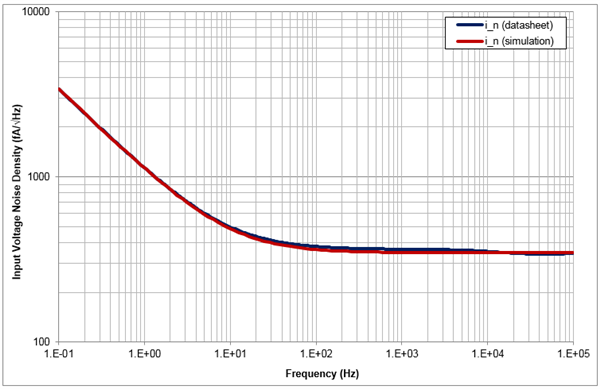Figure 5: OPA1692 in result

Again, the noise characteristic matches the data-sheet curve extremely well.

Total voltage noise

While knowing the input-referred noise of an op amp is useful, it doesn’t paint a complete picture of your circuit’s overall noise performance. A combination of factors like closed-loop gain, bandwidth and the noise contributions of other circuit elements will affect the total amount of noise that appears at the circuit output. Thankfully, most simulators provide a way to measure this type of noise, called total noise or integrated noise, since it’s the integration of all noise sources over the circuit’s effective bandwidth.

Figure 6 shows a more complex op amp circuit, with the OPA1692 configured for a noninverting gain of 10V/V and an additional resistor-capacitor (RC) filter at the output to limit the effective bandwidth to roughly 150kHz.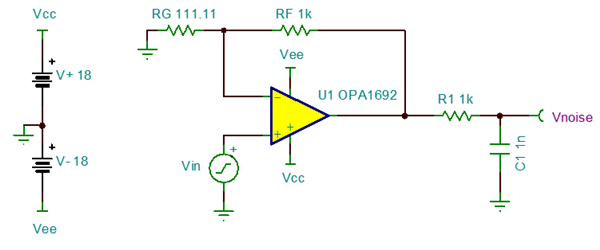Figure 6: OPA1692 total noise example circuit

Run a total noise analysis over a wide frequency range (shown in Figure 7) and measure the noise level at node Vnoise in order to find the total root mean square (RMS) noise, which will appear at the circuit output. You are looking for the level at which the total noise curve flattens out to a constant value at high frequency.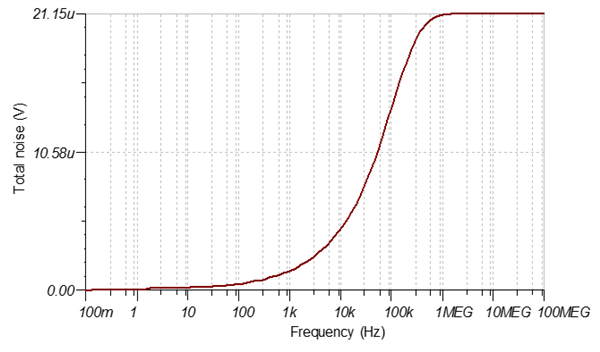Figure 7: OPA1692 total noise result

The test result shows that the total noise of the circuit in Figure 6 is equal to 21.15µVrms, or 126.9µVpp. This is what you would expect to measure if you probed the output of this circuit in the real world. However, keep in mind that the random nature of noise means that the actual noise level may be somewhat higher or lower than what you calculated or simulated. For a deeper discussion, watch the TI Precision Labs – Op Amps video series on noise.

Thanks for reading this sixth and final installment of the “Trust, but verify” blog series! I hope you’ve found the information and techniques in this series useful in your pursuit of more accurate SPICE simulations. If you have any questions about simulation verification, log in and leave a comment, or visit the TI E2E™ Community Simulation Models forum.

• Good article

Some op-amp has build in model for current and voltage noise, I know this becuase I done some experiment with low noise op-amp in the past.

Was is possible to list op-amp that include current/voltage noise generator and how to enable/disable them (for faster spice).

BTW: I use LTSPice because very good spice and editor compare to Tina (I found spice editor very strange and fussy and slow, I gave up using Tina as time waster). Some TI op-amp model did not work well on LTSPice (wondering if this is intended to stop using LTSpice?).

• Hi Richard,

Whether or not the op amp has an included noise generator is not something we can easily list out or filter in parametric search. However, if you download an op amp SPICE model and check its netlist, all the modeled parameters will be given in the header at the top. Disabling them is tricky and would vary depending on the model architecture.

It's true that some of our op amp models don't perform as well in other simulators. However, our newer models are built on an improved and optimized architecture that works well in all PSpice-based simulators.

• Hi Ian,

Thank you for an excellent article.

I disagree with Richard - TINA has proven itself for me and I have simulated every possible circuit one can think of with TINA.

It has not been a "time waster" for me as Richard says.

Please give us more articles such as this.

Kind regards.

• Hi Stephan,

Thank you very much, I'm glad you find TINA and this article useful. Everyone has their favorite simulation software, and each one of them has its strengths and weaknesses. TINA is not perfect, but I believe it has the best math engine behind the scenes, working to find solutions to complex circuits that might fail to converge elsewhere.